# Life Cycle Cost Analysis Example

This example appears in the System Analysis Reference book.

BlockSim Life Cost Analysis Example

Consider the manufacturing line (or system) shown next.The block properties, pool properties and crew properties are given in the following tables. All blocks identified with the same letter have the same properties (i.e., Blocks A = A1 and A2; Blocks B = B1, B2, B3 and B4; and Blocks C = C1, C2, C3 and C4).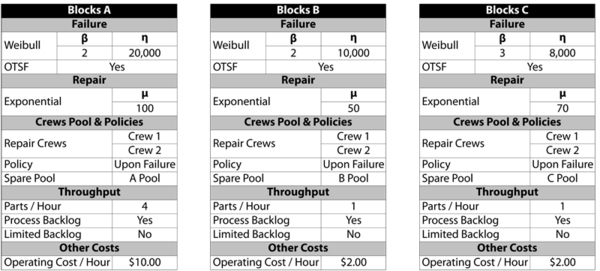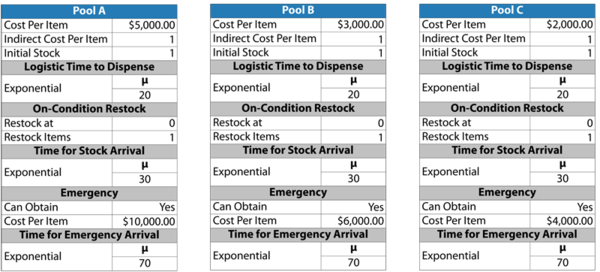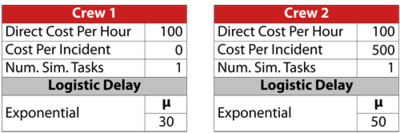This system was analyzed in BlockSim for a period of operation of 8,760 hours, or one year. 10,000 simulations were performed. The system overview is shown next.Most of the variable costs of interest were obtained directly from BlockSim. The next figure shows the overall system costs.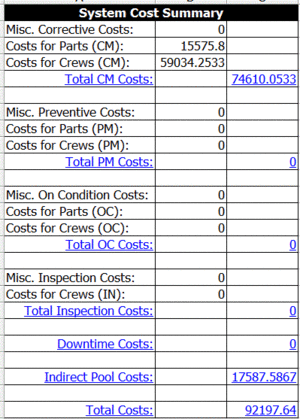From the summary, the total cost is $92,197.64. Note that an additional cost was defined in the problem statement that is not included in the summary. This cost, the operating cost per item per hour of operation, can be obtained by looking at the uptime of each block and then multiplying this by the cost per hour, as shown in the following table. Therefore, the total cost is $92,197+313,813=\406,010.\,\!$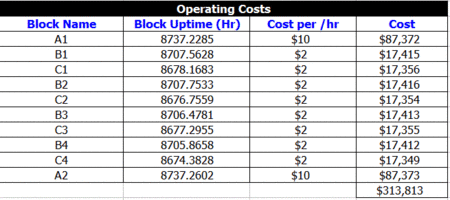If we also assume a revenue of$100 per unit produced, then the total revenue is our throughput multiplied by the per unit revenue, or $31,685\cdot \100=\3,168,500.\,\!$